Nature & Environment

### Become an OU student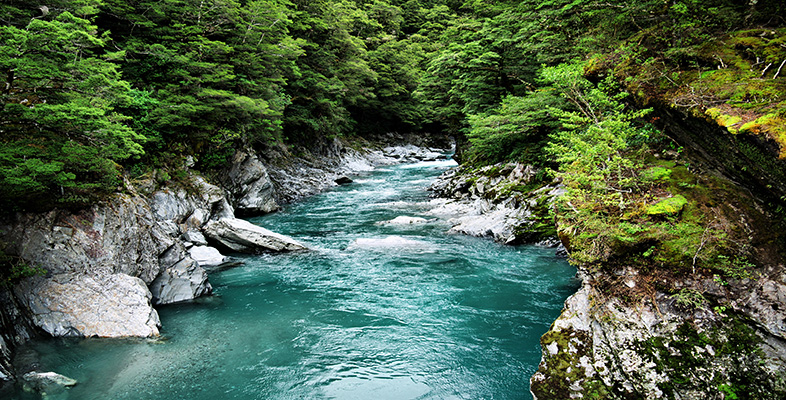Water for life

Start this free course now. Just create an account and sign in. Enrol and complete the course for a free statement of participation or digital badge if available.

# 6.4.1 Writing a chemical equation to describe a chemical process

Natural gas, which is largely methane, is burned to provide heat for cooking and domestic heating and as an industrial power source. This process of burning involves the reaction of methane with oxygen in air to produce carbon dioxide and water.

A chemical equation can be constructed for the reaction of methane with oxygen to give carbon dioxide and water as the products.

1. The first step is to write the formulas of the reactants on the left and the products on the right:

•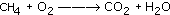2. At this point, all the products and reactants are featured, but the equation is not balanced; the numbers of hydrogen and oxygen atoms are not the same on both sides of the equation. Since oxygen atoms are in pairs in both carbon dioxide and oxygen, you know that you must have an even number of oxygens on the right-hand side of the equation too. To correct this 'odd oxygen' imbalance, add another water molecule to the right-hand side of the equation. Now count up the atoms on both sides of the equations.

•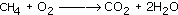There is now one carbon and four hydrogen atoms on each side of the equation. However, there are two oxygen atoms on the left-hand side and a total of 2 + 2 = 4 oxygen atoms on the right.

3. To correct this, add another oxygen molecule on the left-hand side to give the balanced chemical equation:

•The total number of atoms is the same on each side of the equation.

Balancing chemical equations is not always easy but it does come with practice. Try practising by doing Questions 19 and 20 before moving on to the next section, which looks at ions and bonding.

## Question 19

Balance the following equations. All the reactants and products are shown.

1. Magnesium is burned in oxygen to give magnesium oxide: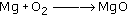2. Carbon and chlorine gas react to form carbon tetrachloride: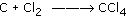3. Potassium oxide is formed by burning potassium in oxygen: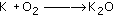4. Hydrogen reacts with chlorine gas to form hydrogen chloride: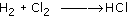Remember that the number of atoms of each element must be the same on both sides of the 'balanced' equation.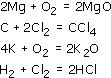Now try writing a balanced chemical equation yourself by doing Question 20.

## Question 20

Write a balanced chemical equation for the chemical reaction in which nitrogen, N2, and hydrogen, H2, react together to give ammonia NH3. These are the only reactants and product involved.

1. The first step is to put the formulas of the reactants on the left and the product on the right.

•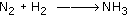2. Now balance the number of atoms of nitrogen. With two atoms of nitrogen on the left, two ammonia molecules are needed on the right. (Remember, N2 means two atoms of nitrogen joined as a molecule.)

•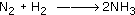3. The next problem is the number of hydrogen atoms: there is a total of six on the right (three in each of the two ammonia molecules) and only two hydrogen atoms in the single hydrogen molecule on the left. To correct this, three hydrogen molecules are needed on the left (to give a total of six atoms).

•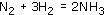The result is a balanced chemical equation for a process in which one molecule of nitrogen reacts with three molecules of hydrogen to give two molecules of ammonia.